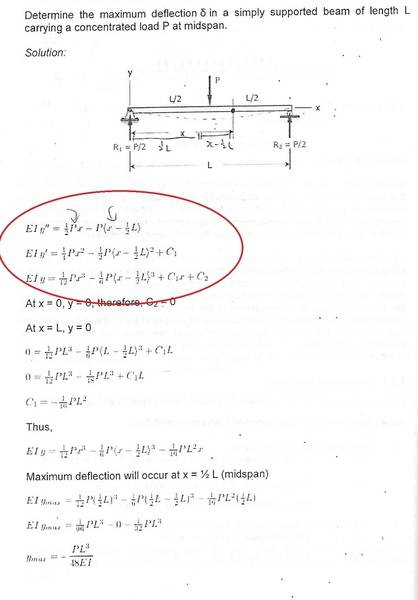# Beam deflection integration help

## Homework Statement

can someone explain about the RHS of EIy' and EIy'' ???
how to get the RHS of EIy" from RHS of EIy' ??
It's not integration of dx , am i right?## The Attempt at a Solution

if it's integration of dx, it should look like this , right?[/B]
EIy' = 0.25P(x^2) - 0.5P(x^2) +0.5PLx ??

SteamKing
Staff Emeritus
Homework Helper

## Homework Statement

can someone explain about the RHS of EIy' and EIy'' ???
how to get the RHS of EIy" from RHS of EIy' ??
It's not integration of dx , am i right?

Why don't you think it's integration to go from EIy" to EIy'? What else would it be?## The Attempt at a Solution

if it's integration of dx, it should look like this , right?[/B]
EIy' = 0.25P(x^2) - 0.5P(x^2) +0.5PLx ??[/QUOTE]

Not necessarily.

Why don't you think it's integration to go from EIy" to EIy'? What else would it be?## The Attempt at a Solution

if it's integration of dx, it should look like this , right?[/B]
EIy' = 0.25P(x^2) - 0.5P(x^2) +0.5PLx ??

Not necessarily.[/QUOTE]

for the EIy" , why shouldnt it = 0.5Px - P(x-0.5L) - 0.5P( L-x) ??

SteamKing
Staff Emeritus
Homework Helper
Not necessarily.

for the EIy" , why shouldnt it = 0.5Px - P(x-0.5L) - 0.5P( L-x) ??[/QUOTE]
It's hard to make out from the image provided, but it appears you start with:

##EIy" = \frac{1}{2} Px - P<x-L>##

Now, the expression P<x-L> usually represents some kind of singularity function, and you don't split up the expression inside the <>.
These expressions usually have some special integration rules which must be followed.

•does the author left out something ? as we can see from the diagram , there are 2 P/2 force at 2 different ends....

Last edited:
SteamKing
Staff Emeritus
Homework Helper
It's hard to make out from the image provided, but it appears you start with:

##EIy" = \frac{1}{2} Px - P<x-L>##

Now, the expression P<x-L> usually represents some kind of singularity function, and you don't split up the expression inside the <>.
These expressions usually have some special integration rules which must be followed.
##EIy" = \frac{1}{2} Px - P<x-L>## this is the author's idea , my idea is = 0.5Px - P(x-0.5L) - 0.5P( L-x)
Which is correct ? the author ? or me ?[/QUOTE]
Obviously, the author is the expert on the interpretation of his own text.

You are not allowed to make up your own mathematics if it does not follow what the author intends.

##EIy" = \frac{1}{2} Px - P<x-L>## this is the author's idea , my idea is = 0.5Px - P(x-0.5L) - 0.5P( L-x)

why the author ignore the moment 0.5P( L-x) ?

for this question, why did C2=0?
in singularity fucntion, <x-a> = 0 only if x<a , and <x-a> =1 if x > a ,
but, in this question, x is drawn beyond 0.5: (a) , am i right?
So, there's also a possibility of <x-a> =1 , am i right?